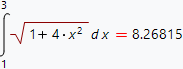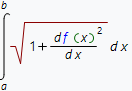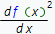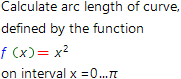# Arc length of decart function curve online calculatorOne application of a definite integral is to calculate the arc length of a plane curve. The figure shows a graph of the function y(x) = x2In order to find out the arc length of the curve of the line shown in the figure, one should calculate the definite integral:More generally, if we have a function y = f(x) defined in Cartesian coordinates and the task is to find the arc length of the curve between the points a and b, we need to calculate the integral:In the above formula, the expressionmeans that you should first calculate the derivative of the function f(x), and then find the square of the resulting expression.

Our online calculator, based of WolframAlpha system, allows one to calculate the length of the curve defined in Cartesian coordinates for any, even very complicated function.

Arc length of decart curve calculatorInput the expression which arclen you want to calculate: